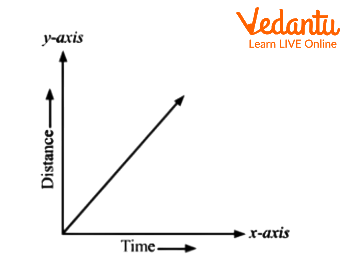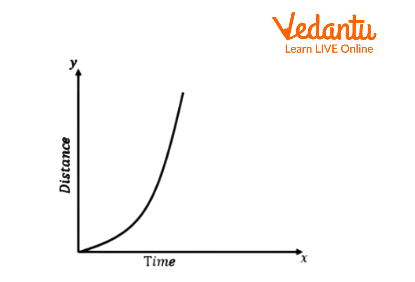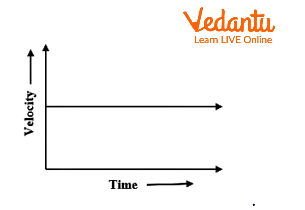Courses
Courses for Kids
Free study material
Free LIVE classes
More

# Graphical Representation of MotionLIVE
Join Vedantu’s FREE Mastercalss

## An Introduction to Motion

When the position of an object changes with time with respect to some reference point, we can say that the object is in motion. The reference point is the point from which the change in position of an object is observed. Graphs offer an easy method to showcase the relationship between two physical quantities.

## Importance of Graphs in Motion

Graphs provide us a convenient method to describe some basic information about a variety of events. The nature of the graph can be studied with the help of the slope. The slope is the inclination that the graph makes with the x-axis. It can also be found out by taking the ratio of change in the y-coordinates to change in the x-coordinates. Graphical representation of motion generally uses graphs and with the help of graphs, we can represent the motion of an object. In the graphical representation of motion, we should take the dependent quantity along the y-axis and the independent quantity along the x-axis.

## Distance-Time Graph

Position of an object with time can be represented by a distance-time graph. The slope of the distance-time graph gives us the speed of an object. Distance-time graphs can be made by considering two situations, either the object moves with a uniform speed or with non-uniform speed. First, we will consider the case of uniform speed. If an object is moving with a uniform speed, we can say that it covers equal distances in equal time intervals. So, we can say that the distance is directly proportional to the time taken.

Suppose, the object covers 10m in the first 5 seconds, it covers another 10m in the next 5 sec and so on. If we mark these points on the graph, the result that we get would be like this:Distance - Time Graph for Uniform Motion

Here, we can see a straight line passing from the origin. Thus, for a uniform speed, the graph of distance travelled against time is a straight line. We can also determine the speed of an object from the distance-time graph. The speed of an object can be determined by calculating the slope of the distance-time graph.

We can plot the distance-time graph for accelerated motion as well. Below is the example of the distance-time graph for non-uniform motion. The nature of this graph shows non-linear variation of the distance travelled by the object with time. So, the below graph shows the motion of an object with non-uniform speed.Distance - Time Graph for Non-Uniform Motion

## Displacement-Time Graph

Displacement is the shortest distance between two points. Displacement-time graph gives us the information of velocity of an object. The rate at which displacement varies with time is known as velocity and the slope of the displacement-time graph gives us the velocity of an object. So, if this graph gives us a straight line which is parallel to the x-axis, then we can say that object is at rest.

## Velocity-Time Graph

The rate at which velocity varies with time is called acceleration and the slope of this graph gives us the information of acceleration of an object. The variation in velocity with time for an object moving in a straight line can be described by a velocity-time graph.Velocity - Time Graph

Here, time and velocity are represented along the x-axis and y-axis, respectively. The graph shows a straight line which is parallel to the x-axis. That means the object has uniform velocity.

## Solved Examples

1. An object is moving along a circular path of radius 7 cm. What is the distance of an object when it completes half revolution.

Ans: Given, radius $r = 7\,cm$

The object is moving along a circular path. We have to calculate the distance travelled by this object when it completes half revolution.

The circumference of the circle is $2\pi r$.

So, here, its distance travelled by it would be $\pi r$.

The distance of an object would be 22 cm when it completes half revolution.

2. A car decreases its speed from $22\,\dfrac{m}{s}$ to $16\,\dfrac{m}{s}$ in 5 sec. Find the acceleration of the car.

Ans: Given, initial speed of the car is $u = 22\,\dfrac{m}{s}$

Final speed of the car $v = 16\,\dfrac{m}{s}$

Time taken $t = 5\sec$

We will use the relation $a = \dfrac{{v - u}}{t}$ to solve this

$\therefore a = \dfrac{{16 - 22}}{5} = - 1.2\,\dfrac{m}{{{s^2}}}$

So, the acceleration of the car is $a = - 1.2\,\dfrac{m}{{{s^2}}}$

## Interesting Facts

• Displacement can be defined from the area under the velocity-time graph.

• The displacement of an object is proportional to the square of time, then we can say that the object moves with uniform acceleration.

• A speedometer is a good example of instantaneous speed.

## Conclusion

Motion can be defined with the help of graphs. We use graphs to describe the motion of an object. In a line graph, we can represent one physical quantity like distance or velocity with another quantity such as time. The different types of graphs for motion help us to understand the speed, velocity, acceleration, and also behaviour of an object.

Last updated date: 17th Sep 2023
Total views: 107.1k
Views today: 2.07k

## FAQs on Graphical Representation of Motion

1. State the difference between speed and velocity.

Here are a few points which show the difference between speed and velocity.

• The speed of the moving object can never be zero and thus the average speed of that object can never be zero. The average velocity of the moving object can be zero when the displacement of the object is zero.

• Speed gives us an idea about how much an object has travelled in a certain period of time but in the case of velocity, it is a measure of the rapidity of the motion along with the direction of the object.

• Speed is a scalar quantity. So, it gives us information about only magnitude. Velocity is a vector quantity, so we can get information about magnitude and direction.

2. Define positive acceleration and negative acceleration.

If the velocity of an object is increasing with time, then we can say that the acceleration of that object is positive. Final velocity of an object will be greater than the initial velocity of an object in case of positive acceleration. The direction of positive acceleration is the same as the direction of motion of the object.

If the velocity of an object is decreasing with time, then we can say that the acceleration of that object will be negative. The direction of negative acceleration will be opposite to the direction of motion of the object. The initial velocity will be greater than the final velocity in case of negative acceleration. Negative acceleration is also called de-acceleration or retardation.

3. State the difference between distance and displacement.

We define distance as the actual path travelled by the object but displacement is the shortest distance between the two points which an object takes. Distance travelled is not a unique path between two points but the displacement refers to a unique path between two points. The distance travelled gives the information of the type of path followed by the object. Displacement between two points gives information only about the initial and the final positions of the object. Distance never decreases with time for a moving object. It can never be zero. Displacement can decrease with time for a moving object.